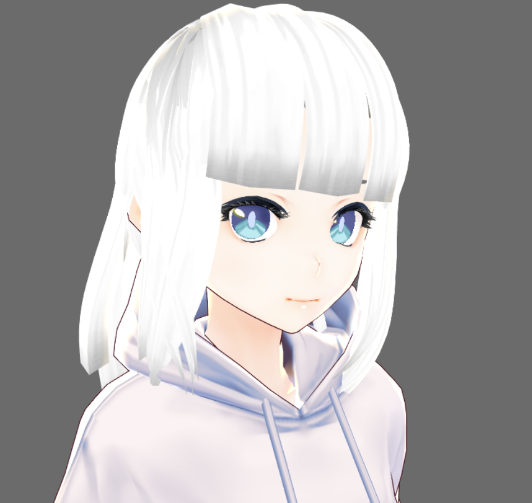Open Vtuber Studio 之開發(1)Toby
Toby

## 由頭部開始

### Face-api.js

FaceAPI.js 是一個使用 Neural Network （神經網絡）而編寫的臉部捕捉 API。話雖如此，它卻內置了很多不同的 Network Model （模型）來做同一件事情。而在這個開源計劃中我看上了這個功能：Face Landmark Detection

### 動態捕捉及 VRM 模型動作 映射演算法

1. 第 1點跟第 17點之間的距離除了前後移動之外基本上不會改變
2. 第 1點跟第 9點之間的垂直距離會跟據你頭部上下望而產生線性變化
3. 第在第 3跟 15點間畫一條直線，在頭部旋轉的時候第 31點會按比例的在這條直線上滑動

### 自動眨眼

``````function createEyeBlinkBlendValueFromCycle(s){
//Eclipse time to sin function
const scaleRatio = 2;
const eyeCloseIntervalScale = 0.18;
return 0;
}else{
}

s = Math.sin( Math.PI * eyeBlinkingTimer * scaleRatio);
s = s * 3;
var baseFormula = Math.min(s, 1);
if (baseFormula < 0){
}else if (s > 1){
//Eye closed. Give it a blink interval skip
}
return baseFormula;
}else{
return 0;
}
}``````

``````function blinkEye(){
setTimeout(function(){
},getRandomInt(2000,10000));
}
function getRandomInt(min, max) {
min = Math.ceil(min);
max = Math.floor(max);
return Math.floor(Math.random() * (max - min + 1)) + min;
}``````